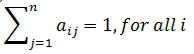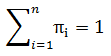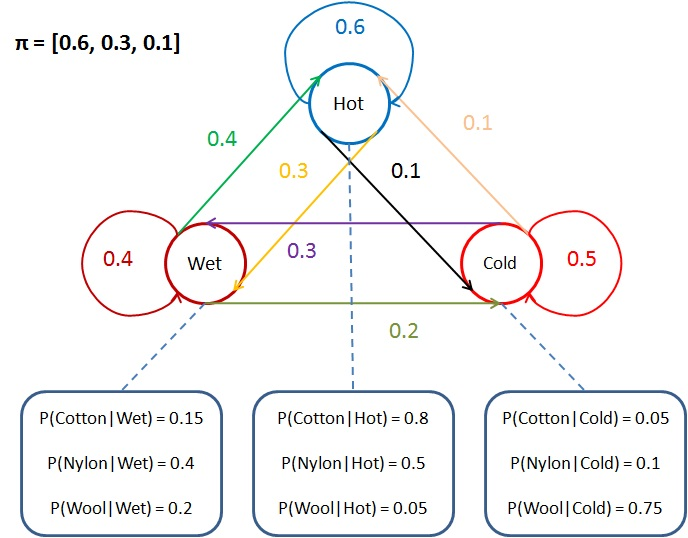Please visit, subscribe and share 10 Minutes Lectures in Computer Science

## Hidden Markov Model

Hidden Markov Model (HMM) is a simple sequence labeling model. It is a statistical Markov model in which the system being modeled is assumed to be a Markov process with unobserved (i.e. hidden) states. By relating the observed events (Example - words in a sentence) with the hidden states (Example - part of speech tags), it helps us in finding the most probable hidden state sequence (Example – most relevant POS tag sequence for the given input sentence).
HMM can be defined formally as a 5-tuple (Q, A, O, B, π) where each component can be defined as follows;
 Component Detailed components Description Q q1, q2, q3, …, qN Set of N hidden states A a11, a12, …, ann Set of transition probabilities A is the state transition probability matrix Each aij in A represents a transition probability value of moving from state i to state j. Sum of transition probability values from a single state to all other states should be 1. That is,O o1, o2, …, oT A sequence of T observations B bi(ot) A sequence of observation likelihoods (emission probabilities) Each bi(ot) represents the emission probability. That is, the probability of an observation ot which  is generated from a state i. π π1, π2, …, πN Set of initial probabilities. π1 is the probability that the Markov chain will start in state i. if π1 = 0, it implies that the state i cannot be an initial state. The sum of all initial probabilities should be 1. That is,### Understanding Hidden Markov Model - Example:

These components are explained with the following HMM. In this example, the states are related to the weather conditions (Hot, Wet, Cold) and observations are related to the fabrics that we wear (Cotton, Nylon, Wool).As per the given HMM,
• Q = set of states = {Hot, Wet, Cold}

• A = transition probability matrix
o   Transition probability matrix
 Current state Previous state Hot Wet Cold Hot 0.6 0.3 0.1 Wet 0.4 0.4 0.2 Cold 0.1 0.4 0.5
o   How to read this matrix? In this matrix, for example, aij is a transition probability from state i to state j [which is represented as conditional probability P(j|i)];
aij = a11 = P(Hot|Hot) = 0.6
aij = a23 = P(Cold|Wet) = 0.2
aij = a31 = P(Hot|Cold) = 0.1
o   Sum of transition probability from a single state to all the other states = 1. In other words, we would say that the total weights of arcs (or edges) going out of a state should be equal to 1. In our example;
P(Hot|Hot)+P(Wet|Hot)+P(Cold|Hot) = 0.6+0.3+0.1 = 1

• O = sequence of observations = {Cotton, Nylon, Wool}
• B = Emission probability matrix
o   Emission probability matrix
 Cotton Nylon Wool Hot 0.8 0.5 0.05 Wet 0.15 0.4 0.2 Cold 0.05 0.1 0.75
o   The above said matrix consists of emission probability values represented as bi(ot). bi(ot) is the probability of an observation ot generated from a state bi.  For example, P(Nylon | Hot) = 0.5, P(Wool | Cold) = 0.75 etc.
• π = [π1, π2, …, πN] = set of prior probabilities = [0.6, 0.3, 0.1]. Here, the values refer to the prior probabilities P(Hot) = 0.6, P(Wet) = 0.3, and P(Cold) = 0.1

**********